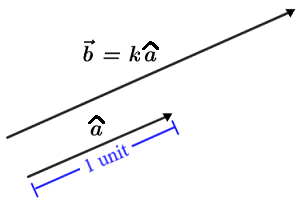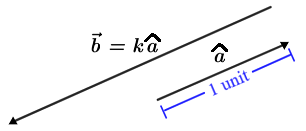# Unit vector

A unit vector is a vector that has a magnitude of 1 unit. Unit vectors are typically denoted using a lower case letter with a circumflex ("hat") symbol above, for example:.

A unit vector can be scaled such that it forms a vector whose magnitude is some scalar multiple of the unit vector. When multiplied by a scalar, the magnitude of the unit vector changes, but its direction is preserved unless the scalar is negative; if the scalar is negative, the direction of the new vector points in the opposite direction of the unit vector, as shown in the figure below.Positive k Negative k

The magnitude of the vectorformed by multiplying a unit vectorby a scalar k, is denoted as follows:## How to find the unit vector of a given vector

To find the unit vector of a given vector, divide by the magnitude (or norm) of the vector. The unit vector,, for, is,

where the magnitude. The process of finding a unit vector is sometimes referred to as normalizing the vector.

Example

Find the unit vector for.

First find the magnitude of v:Then the unit vector is:To confirm that this is the unit vector, compute the magnitude:## Standard unit vectors

The standard unit vectors are the unit vectors parallel to the axes of the coordinate system. In the 2D coordinate system, the standard unit vectors can be written as. Any vector in the 2D coordinate plane can be written as a linear combination of i and j. For example, we can writeas:In general form, given, then:,

where v1 and v2 are scalars.

Example

Let vector v have its tail at (3, 5) and head at (-2, 1). Write v as a linear combination of i and j.

The components of v areor in column formwhich can be written as a linear combination of i and j as follows:The vector is shown in the figure below:

It is worth noting that the standard unit vectors in 2D or any other dimension are independent of each other, meaning that they cannot be written as a linear combination of any other vectors in that particular dimension.

In the 3D coordinate plane, the standard unit vectors are:The figure below shows vectors i, j, and k graphed on the 3D coordinate plane along with w, a vector that is a linear combination of i, j, and k.

## Writing parallel vectors with unit vectors

Unit vectors can be used to write parallel vectors. Two vectors are parallel if one can be written as a scalar multiple of the other. As such, unit vectors can be used to write any number of parallel vectors.

Example

Write vector w in component form given that it has a magnitude of 5 units and is parallel toFirst, find the unit vector for v:Sincehas unit length and is pointed in the same direction as v, it is parallel to v, so we can write w as a scalar multiple ofsuch that. Thus:## Using unit vectors and direction angles to write vectors

Ifis a column vector,is its unit vector, and θ is the angle between the x-axis and, then the components oflie on the unit circle, and we can use the cosine and sine functions to determine its components such thatsince. Refer to the figure below.

Since θ determines the direction of, it is often referred to as the direction angle for. Vector v has the same direction angle as, so we can write v as,

or as a linear combination:The direction angle can then be determined using the components of v and the tangent function:Thus,, where an adjustment for θ may need to be made depending on what quadrant the head of v is in.

Example

Write vector v as a linear combination of vectors i and j given that it has a magnitude of 6 units and a direction angle of 30°.

Using,Vector v is graphed in the figure below: